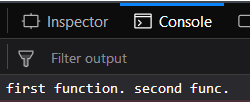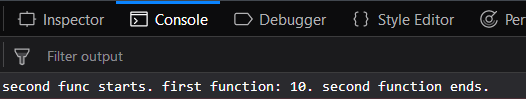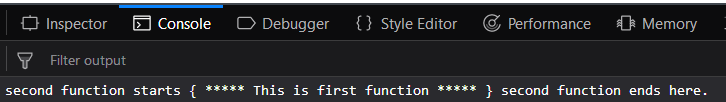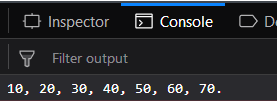# Underscore.js | _.wrap() with Examples

The Underscore.js is a JavaScript library that provides a lot of useful functions like the map, filter, invoke etc even without using any built-in objects.
The _.wrap() is used to wrap a function inside other function. It means that the first calling function (a function which is calling another function in its body) is called and then the called function is being executed. If the calling function does not call the called function then the second function will not be executed.

Syntax:

`_.wrap( function, wrapper )`

Parameters: This function accepts two parameters which are listed below:

• function: This parameter is used to hold the function name.
• wrapper: This parameter is used to hold the wrapper function which wrap first function.

Return value: It returns the output of both the function involved in the process.

Passing no arguments of both functions of _.wrap() function: The function is called which is passed to the console.log() function. That function body contains the _.wrap() function which calls the other function. Then the other function body gets executed and finally the control returns to the called function.

Example:

 ` ` `<``html``> ` `     `  `<``head``> ` `    ``<``script` `src` `=  ` `"https://cdnjs.cloudflare.com/ajax/libs/underscore.js/1.9.1/underscore-min.js"` `> ` `    `` ` ` ` ` `  `<``body``> ` `    ``<``script` `type` `= ``"text/javascript"``> ` `        ``var func1 = function() { return "first function. "; }; ` `         `  `        ``func2 = _.wrap(func1, function(func) { ` `            ``return func() + "second func. "; ` `        ``}); ` `         `  `        ``console.log(func2()); ` `    `` ` ` ` ` `  `                     `

Output:Passing an argument to the second function of _.wrap() function: Passing the argument to the second function from the first function definition. In the definition, the parameter to the second function will be passed by giving the parameter inside double quotes (” “) inside the brackets. The function will work as earlier like first, the first function which is called will be executed and then the first function will be executed.

Example:

 ` ` `<``html``> ` ` `  `<``head``> ` `    ``<``script` `src` `=  ` `"https://cdnjs.cloudflare.com/ajax/libs/underscore.js/1.9.1/underscore-min.js"` `> ` `    `` ` ` ` ` `  `<``body``> ` `    ``<``script` `type``=``"text/javascript"``> ` `        ``var func1 = function(a) {  ` `            ``return "first function: " + a + ".";  ` `        ``}; ` `         `  `        ``func2 = _.wrap(func1, function(func) { ` `            ``return "second func starts. "  ` `            ``+ func("10") + " second function ends."; ` `        ``}); ` `         `  `        ``console.log(func2()); ` `    `` ` ` ` ` `  `                     `

Output:Passing special characters as argument to the second function of _.wrap() function: Passing special characters to the second function then the _.wrap() function will work in the same way. It takes the special character as a normal character and then works in the same way. The first function will be called and then it’s body will be executed. After that when the first function is called inside the second function, the second function body will get executed.

Example:

 ` ` `<``html``> ` ` `  `<``head``> ` `    ``<``script` `src` `=  ` `"https://cdnjs.cloudflare.com/ajax/libs/underscore.js/1.9.1/underscore-min.js"` `> ` `    `` ` ` ` ` `  `<``body``> ` `    ``<``script` `type``=``"text/javascript"``> ` `        ``var func1 = function(a) { return a; }; ` `     `  `        ``func2 = _.wrap(func1, function(func) { ` `            ``return "second function starts { "  ` `            ``+ func("***** This is first function *****")  ` `            ``+ " } second function ends here."; ` `        ``}); ` `         `  `        ``console.log(func2()); ` `    `` ` ` ` ` `  `                     `

Output:Passing numbers as arguments to the second function of the _.wrap() function: The second function will also consider the numbers in the same way as they consider the characters. This will give the output accordingly.

Example:

 ` ` `<``html``> ` ` `  `<``head``> ` `    ``<``script` `src` `=  ` `"https://cdnjs.cloudflare.com/ajax/libs/underscore.js/1.9.1/underscore-min.js"` `> ` `    `` ` ` ` ` `  `<``body``> ` `    ``<``script` `type` `= ``"text/javascript"``> ` `        ``var func1 = function(a) { return a + ", "; }; ` `         `  `        ``func2 = _.wrap(func1, function(func) { ` `            ``return "10, 20, " + func("30, 40, 50") + " 60, 70."; ` `        ``}); ` `         `  `        ``console.log(func2()); ` `    `` ` ` ` ` `  `                     `

Output:Note: These commands will not work in Google console or in Firefox as for these additional files need to be added which they didn’t have added. So, add the given links to your HTML file and then run them.

 `  `My Personal Notes arrow_drop_upCheck out this Author's contributed articles.

If you like GeeksforGeeks and would like to contribute, you can also write an article using contribute.geeksforgeeks.org or mail your article to contribute@geeksforgeeks.org. See your article appearing on the GeeksforGeeks main page and help other Geeks.

Please Improve this article if you find anything incorrect by clicking on the "Improve Article" button below.

Improved By : Akanksha_Rai